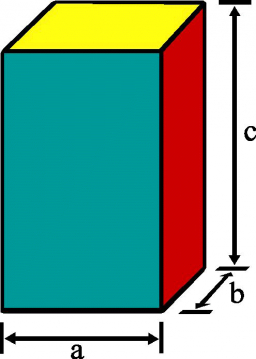The surface of the quadrangular block is 3.4 m2. The edges of the figure are 8cm and 10cm long. Calculate the volume of the prism.

V =  400 cm3

### Step-by-step explanation:Did you find an error or inaccuracy? Feel free to write us. Thank you!

Tips to related online calculators
Need help to calculate sum, simplify or multiply fractions? Try our fraction calculator.